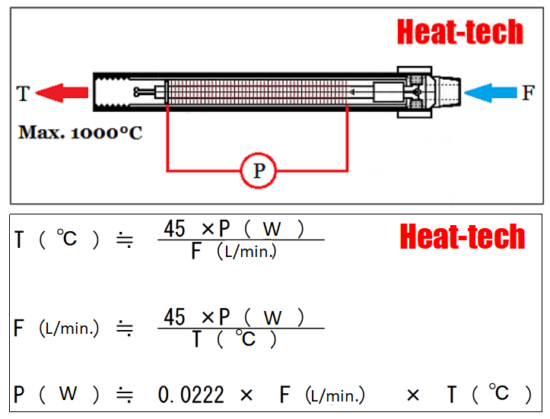Home / Air Blow Heater / The guide to the Air Blow Heater / Chapter 1.Basic knowledge of Air Blow Heater / 1-10.Calculation Method for Selecting the Model of the Air Blow Heater

# 1-10.Calculation Method for Selecting the Model of the Air Blow HeaterThe temperature of the hot air from theAir Blow Heater is T [℃], the gas flow rate is F [L / min.], And the power consumption of the Air Blow Heater is P [w].

Please be sure to double the safety factor before selecting the power consumption of the Air Blow calculated from the formula.
Example) When calculating P (electric power)

P (electric power) ≒ 0.0222 x 200 L / m x 600 ℃ = 2664W x 2 (safety factor) = 5328W

In order to raise T (temperature), F (flow rate) should be reduced. However, if the hot air temperature exceeds 1000 ℃, it will overheat and the wire will break, so be sure to set temperature of hot air to 1000 ℃or less.
If F (flow rate) cannot be changed, T (temperature) can be increased by increasing P (electric power) of the Air Blow Heater.

If it is smaller than the rated power of the Air Blow Heater selected by P, it can be dealt with by lowering the supply voltage. The method of controlling the voltage is as described above.
* The above formula ignores thermal efficiency, but it is necessary to consider thermal loss. Normally, the thermal efficiency is about 90% to 80%, but when the temperature is high and the flow rate is low, the heat loss increases and the thermal efficiency decreases, so in the case of a low flow rate, the thermal efficiency can be 50% to 60%. On the contrary, in the case of a large flow rate, the thermal efficiency becomes 90% or more.
* Regarding the capacity of the Air Blow Heater, we have a track record of manufacturing in the range of 30w to 70.000w. In addition, we will manufacture any custom specifications if possible.

### How to select an Air Blow Heater from the wind speed

Example) Cross section is 10mm □ I want hot air at 100 ℃ at a wind speed of 15m in a pipe. Which Air Blow Heater should I choose?

① First, align the units. Here, it is unified to mm.
Cross section 10mm × 10mm = 100mm ^ 2
Since the wind speed and flow velocity are every second, 15m / sec should be 15000mm / sec.
(2) The flow rate F can be calculated by multiplying the cross section by the wind speed.
Flow rate F = 100mm ^ 2 × 15000mm / sec = 1500000mm ^ 3 / sec
Since the flow rate F is usually expressed in L / min,
1L = 1000000mm ^ 3
F = 1500000mm ^ 3 / sec = 1.5L / sec
F = 1.5L / sec x60 = 90L / min.
(3) The formula for calculating the required power WP from the flow rate F and the temperature rise T is
Required power WP = 0.0222 × F × T
F = 90L / min. T = 100 ℃, so
Required power WP = 0.02 × 90 × 100 ≒ 200w
④ Correct with the safety factor SR.
The safety factor SR is 200%.
Power SP = 200w ÷ 2.0 = 400w
A ABH100V-450W / □□ or ABH200V-450W / □□ is Air Blow Heater heater that meets the specifications.

### How to select an Air Blow Heater based on air volume and temperature

Please select by multiplying the number of output watts in the table below by the safety factor of 200%.